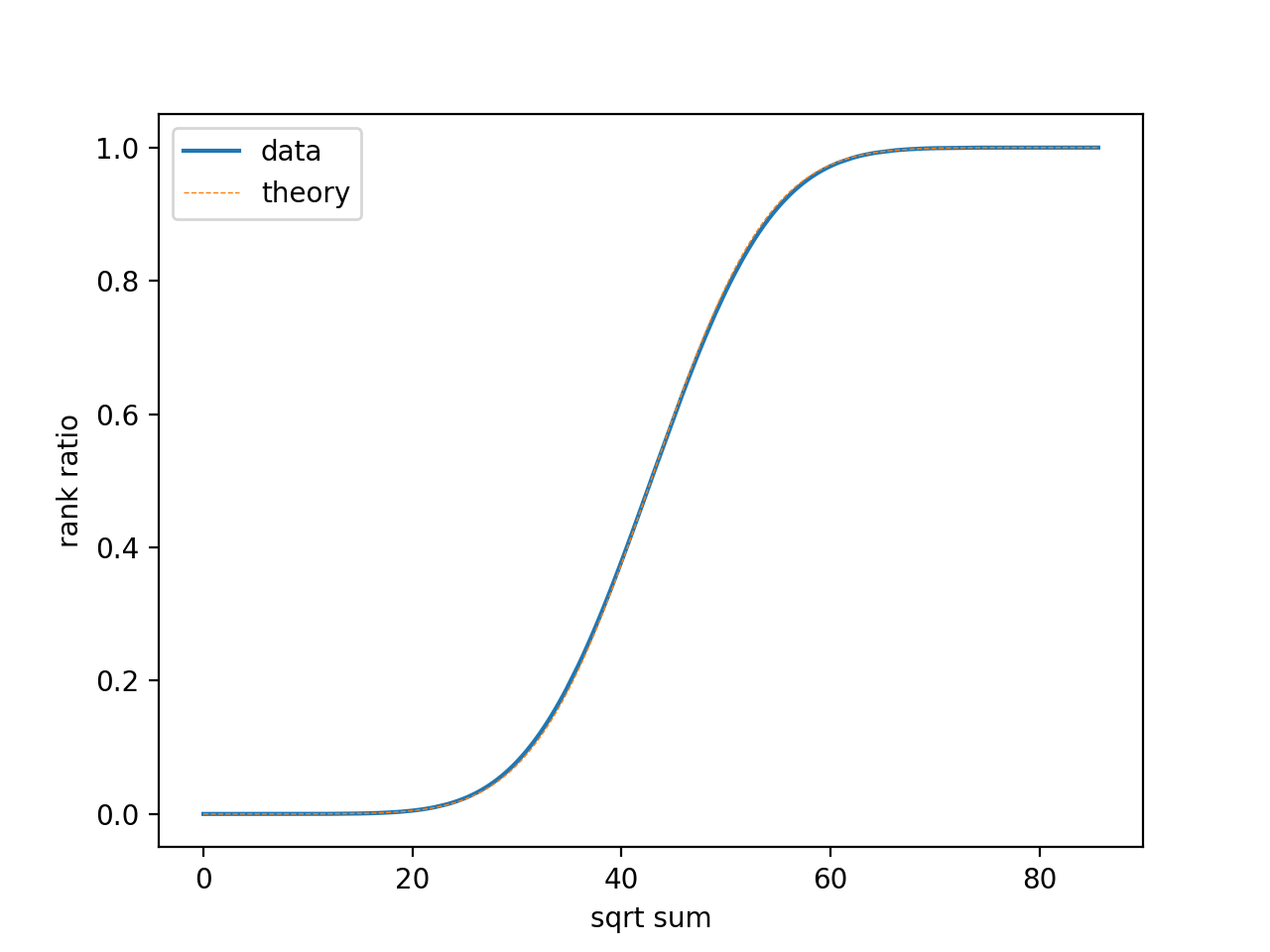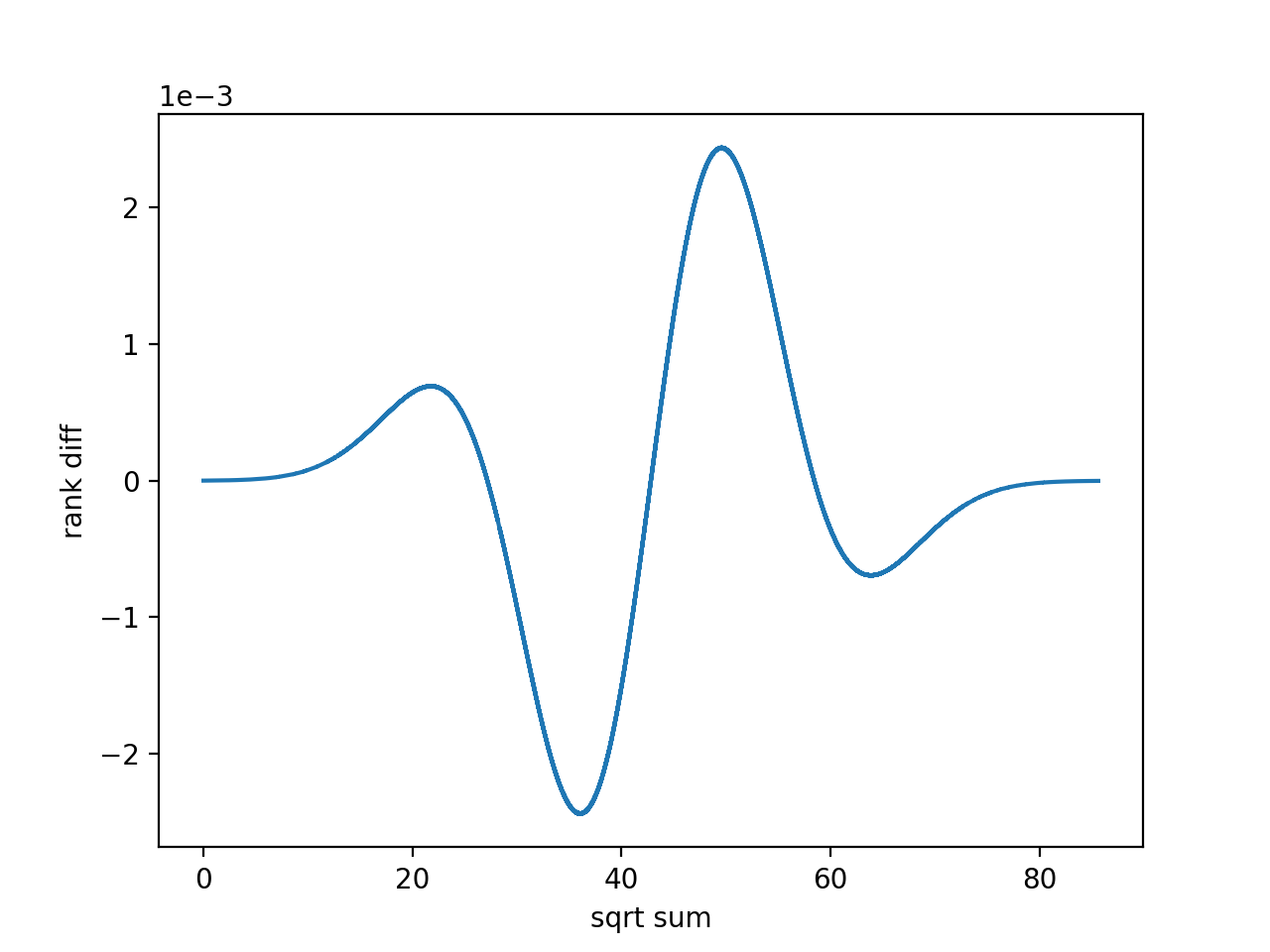# 一个特殊子集和问题, 以及指数复杂度下常数优化的意义

2 分钟阅读

2019 年 12 月 03 日

$\sqrt 1, \sqrt 2, \cdots, \sqrt{50}$ 这 50 个数分成两部分, 要求两部分的和最接近. 问这两个和的差最小是多少.

## 解析分析

(2019-12-07 update: 这个证明数学上是错误的. CLT 在数学上误差项是对于累积分布函数的估计 (Berry-Esseen thm), 收敛速度达不到 $O(2^N)$ 的话就没有办法应用在这个场景里. 一个更直观的论证是, CLT 在 i.i.d 下总是有效, 但把根号和改成 i.i.d. 给出 $O(1)$ 的误差. )

Lyapunov CLT 的条件, 对于任意的 $\delta > 0$ 都是成立的. 上面定义的随机变量 $X\sim N(\mu, \sigma^2)$ 有 ($N=50$):

CLT 的应用, 对于 50 个数的和, 收敛小于 $\sqrt N$. 另外第一个样本出现是一个概率估计, 所以实际上的结果应该比这个数字大一个 $O(1)$ 的系数. 后文的分析显示确实只有大约 2 倍.

## 程序计算

1. 取其中 25 个数, 计算所有的子集和 $s_i$ 并排序, $O(n\log n)$
2. 取余下的 25 个数, 计算子集和 $s’_i$ 并在上一步得到的序列中二分查找 $s_j$ 使得 $s’_i + s_j$ 最接近全体和的一半, $O(n\log n)$
3. 记录上述结果的最小值

1. 不要看到指数复杂度就止步不前. 指数意味着对不太大的问题规模, 计算量就会很大, 但也意味着常数项的优化是显著的.
2. OS 对于栈内存的使用是有限制的. 在这个程序中如果 $2^{25}$ 个数作为静态内存写入程序的话, 会导致段错误 (x64 Linux/gcc)
3. 练习了快排以及求取最大值的并行化

2020-01-07 更新 闲来无事画了个图, 把 1,2,…,25 的平方根求和做了个图, 如下